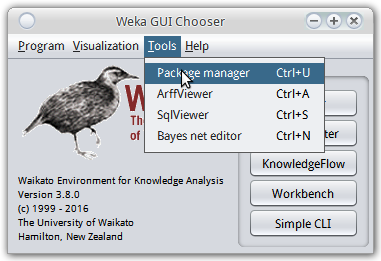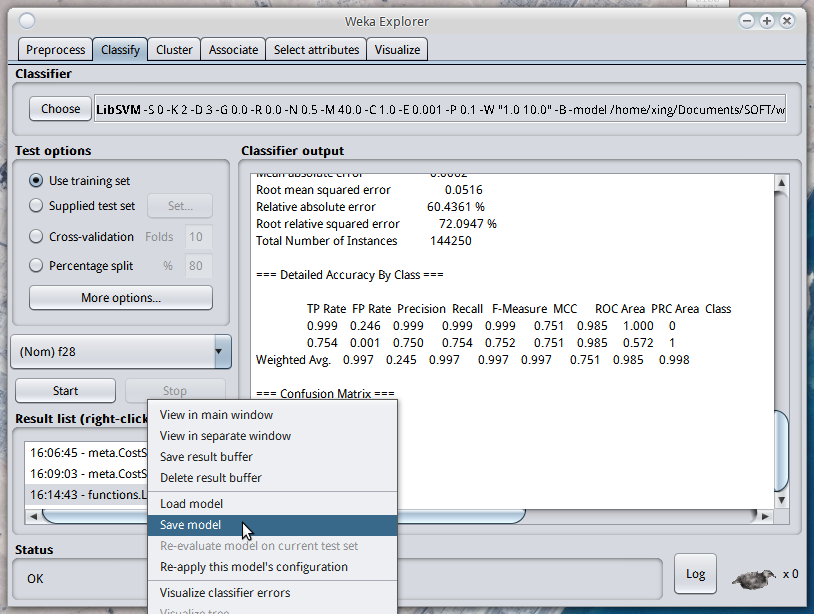[TOC]

# 1. Include jars into project

weka官网的下载链接里选择linux版本的weka压缩包即可, 下载以后找到weka.jar文件, 在工程里将其include一下就可以使用了(btw, 现在开始放弃eclipse, 进入IDEA的怀抱了...).

weka的文档在解压缩的文件里有, 另外在线文档在: http://weka.sourceforge.net/doc.stable-3-8/

### about libsvm...# 2. terminology

• `Instances`: 就是dataset, 比如training set或者test set, Instances实际上就是一个Instance的集合
• `Instance`: 就是一个数据点了
• `Attribute`: 一个数据点有一些attribute (别处一般叫做feature), 其中有一个attribute其实是label(可以为missing)
• `Classifier`: weka里的Classifer其实是也包含了regressor或者cluster... 后面都称之为model
• `Evaluation`: 给定一个model和一个dataset, 给出evaluation的数据, 类似GUI界面给出的那些内容

# 3. 在程序里构建数据

### 新建Attribute

http://weka.sourceforge.net/doc.stable-3-8/weka/core/Attribute.html

```// Create numeric attributes "length" and "weight"
Attribute length = new Attribute("length");
Attribute weight = new Attribute("weight");
```

```// Create list to hold nominal values "first", "second", "third"
List my_nominal_values = new ArrayList(3);
my_nominal_values.add("first");
my_nominal_values.add("second");
my_nominal_values.add("third");
// Create nominal attribute "position"
Attribute position = new Attribute("position", my_nominal_values);
```

### 新建Instances(dataset)

http://weka.sourceforge.net/doc.stable-3-8/weka/core/Instances.html
`Instances`实际上就是一个`Instance`的集合, Instances可以类比为pandas里面的DataFrame, 然后每个instance相当于一行. 另外`Instances``Instance`多的就是Attribute信息(类比为pandas里DataFrame的表头head).

`Instances`的构造函数有两种, 一种是直接在arff文件里读取, 这个后面再说. 另一种构造函数是在java函数里构建Instance时用的, 它构造一个空的Instance集合, 构造函数提供dataset的名字, attribute的集合(arraylist)以及初始的capacity:

`Instances(String name, ArrayList<Attribute> attInfo, int capacity)`

```ArrayList<Attribute> atts = new ArrayList<Attribute>();
atts.add(length);
atts.add(weight);
atts.add(position);
Instances adataset = new Instances("aDataSet", atts, 10);
```

(上面代码里的`length`, `weight``position`都是前面声明的Attribute对象)

Instances还可以指定哪一列对应的是class label (单个Instance则不能 — 因为单个Instance并没有表头信息attInfo):

`void setClassIndex(int classIndex)`

### 新建Instance

http://weka.sourceforge.net/doc.stable-3-8/weka/core/Instance.html
`Instance`是一个接口而不是一个类, 一半常用的是`DenseInstance`类(它又继承自`AbstractInstance`抽象类)

`void setDataset(Instances instances)`

```// Create empty instance with three attribute values
Instance inst = new DenseInstance(3);
instance.setDataset(  adataset); // before calling setValue, should first set the Dataset!``
// Set instance's values for the attributes "length", "weight", and "position"
inst.setValue(length, 5.3);
inst.setValue(weight, 300);
inst.setValue(position, "first");
```

# 4. 在程序里训练model

http://weka.sourceforge.net/doc.stable-3-8/weka/classifiers/Classifier.html

Classifier是一个Interface, 可以在文档里看到有很多类都实现了这个interface, 主要是三个常用的函数:

• `void buildClassifier(Instances data)` : 用data数据进行训练
• `double classifyInstance(Instance instance)` : 预测一个Instance的label
• `double[] distributionForInstance(Instance instance)` :对于每一个可能的类, 给一个probability, 返回一个double数组

# 5. 从文件读入数据和model(参考链接: https://weka.wikispaces.com/Serialization 以及 https://weka.wikispaces.com/Use+Weka+in+your+Java+code)

```Classifier clf = (Classifier) weka.core.SerializationHelper.read("/some/where/j48.model");
Instances testset = new Instances(new BufferedReader(new FileReader("/some/where/test.arff")));``
```

# 6. 输出Evaluation统计

http://weka.sourceforge.net/doc.stable-3-8/weka/classifiers/evaluation/Evaluation.html
Evaluation的构造函数里提供的Instances应该为training set, 这个训练集的作用是"to get some header information and prior class distribution information", 如果构造时给的是testing set的话, 应该调用`useNoPriors()`函数一下.

```Instances trainInstances = ... instances got from somewhere
Instances testInstances = ... instances got from somewhere
Classifier scheme = ... scheme got from somewhere

Evaluation evaluation = new Evaluation(trainInstances);
evaluation.evaluateModel(scheme, testInstances);
System.out.println(evaluation.toSummaryString());
System.out.println(evaluation.toMatrixString());
```

# 7. 输出预测结果

Voila, 大概就是这样, weka这个工具还是蛮好用的(只要能忍受它界面的丑), 而且也算没有太多的坑...

comments powered by Disqus